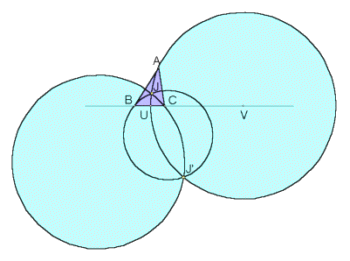# ISODYNAMIC POINTS

 Suppose ABC is a triangle. Let U and V be the points on sideline BC met by the interior and exterior bisectors of angle A. The circle having diameter UV is called the A-Apollonian circle. The B- and C- Apollonian circles are likewise constructed. The three circles meet in two points, labeled J and J', called the isodynamic points.Specifically, the point with trilinears

sin(A + pi/3):
sin(B + pi/3):
sin(C + pi/3)

is the first isodynamic point, and the point with trilinears

sin(A - pi/3):
sin(B - pi/3):
sin(C - pi/3)

is the second isodynamic point. Both points are discussed in

Roger A. Johnson, Advanced Euclidean Geometry, Dover Publications, Mineola, NY, 1960, pp. 294-297.